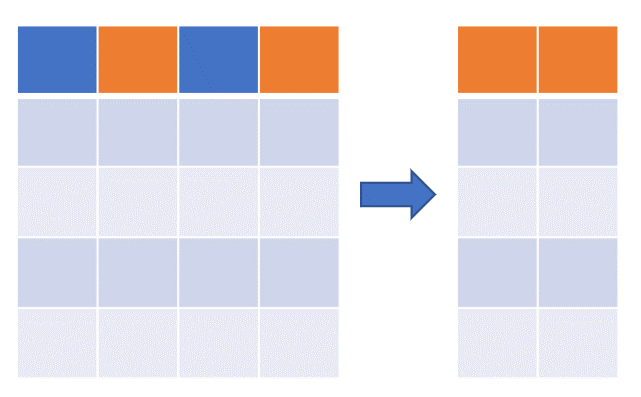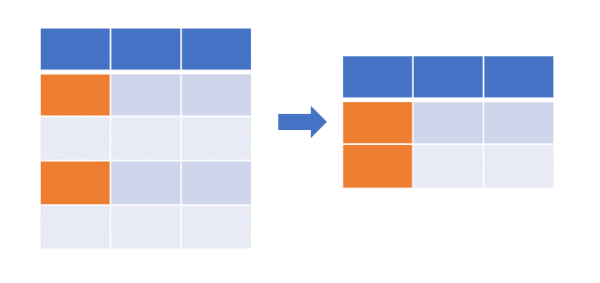# Subset Data Frames in R

Subsetting or filtering the Data Frames is an essential part of data analysis. In this article, you will learn how to subset Data Frames in R.

First, create a sample Data Frame

``````df <- data.frame(col1 = c("A", "B", "C", "D", "E"),
col2 = c(1, 2, 3, 4, 5),
col3 = c(0.1, 0.2, 0.3, 0.4, 0.5),
col4 = c("abc", "def", "xyz", "lmn", "pqr"))

df
# output
col1 col2 col3 col4
1    A    1  0.1  abc
2    B    2  0.2  def
3    C    3  0.3  xyz
4    D    4  0.4  lmn
5    E    5  0.5  pqr
``````

### Subset Data Frame based on columnsThe Data Frame `df` contains four columns (`col1`, `col2`, `col3`, `col4`). Subset `df` to contain only `col2` and `col4` columns,

Using column indexes,

``````df[, c(2, 4)]

# output
col2 col4
1    1  abc
2    2  def
3    3  xyz
4    4  lmn
5    5  pqr
``````

Using column names,

``````df[, c("col2", "col4")]

# output
col2 col4
1    1  abc
2    2  def
3    3  xyz
4    4  lmn
5    5  pqr
``````

You can also use `subset` function to subset Data Frame,

``````subset(df, select=c(2, 4))
# output
col2 col4
1    1  abc
2    2  def
3    3  xyz
4    4  lmn
5    5  pqr
``````

You can also use `select` function from dplyr package to subset Data Frame,

``````library(dplyr)
df %>% select(col2, col4)
# output
col2 col4
1    1  abc
2    2  def
3    3  xyz
4    4  lmn
5    5  pqr
``````

### Subset Data Frame based on rowsThe Data Frame `df` contains five rows. Subset `df` to contain required rows,

Using row indexes,

``````df[c(1, 3), ]

# output
col1 col2 col3 col4
1    A    1  0.1  abc
3    C    3  0.3  xyz
``````

Using row ranges,

``````df[c(1:3), ]

# output
col1 col2 col3 col4
1    A    1  0.1  abc
2    B    2  0.2  def
3    C    3  0.3  xyz
``````

Select random rows (in non-sequential order)

``````df[c(1, 3:5), ]
# output
col1 col2 col3 col4
1    A    1  0.1  abc
3    C    3  0.3  xyz
4    D    4  0.4  lmn
5    E    5  0.5  pqr
``````

### Subset Data Frame from Data FrameSelect rows and columns (a subset of DataFrame) using row and column indexes,

``````df[1:3, 2:3]
# output
col2 col3
1    1  0.1
2    2  0.2
3    3  0.3
``````

## Enhance your skills with courses on R

If you have any questions, comments or recommendations, please email me at reneshbe@gmail.com